# ISEE Middle Level Math : How to divide variables

## Example Questions

← Previous 1 3

### Example Question #1 : How To Divide Variables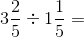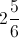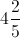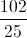Explanation:

First convert each mixed number into and improper fraction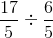Then convert the operation to multiplication and flip the second fraction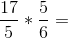Reduce where possible and multiply to solve: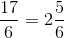The answer is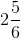### Example Question #2 : How To Divide Variables

Simplify: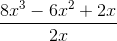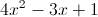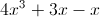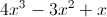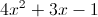Explanation: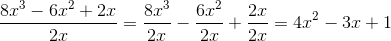### Example Question #3 : How To Divide Variables

Solve for B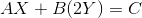Not enough information provided.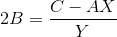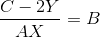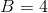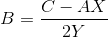Explanation:

This problem looks strange since it mostly contains variables, in particular unknown variables. Still remember the order of operations: parentheses, exponents, multiplication, division, addition, and subtraction. We still use those operations in this case. All we must do is move the variables around to solve for.

In this case we can subtract (additive inverse)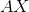to the other side first.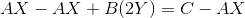Rewrite our problem.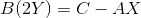Stop for a moment and look at the progress. Go back to the original question, solve for. Our problem showsabout to be multiplied to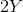, but we do not necessarily have to multiply because we can divide byto both sides of the equation, which would save us a step as well as have B by itself.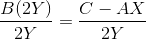### Example Question #1 : How To Divide Variables

Suppose you know the values of, and, and you want to evaluate the expression below.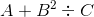Which of the following is the first step you must complete?

Evaluate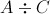.

Evaluate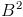.

Evaluate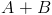.

Evaluate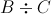.

Evaluate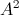.

Evaluate.

Explanation:

Use the order of operations, PEMDAS: Parentheses, Exponents, Multiplication, Division, Addition, Subtraction

In our expression, there are no parentheses, so squarefirst.

### Example Question #1231 : Hspt Mathematics

Divide: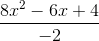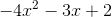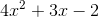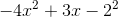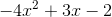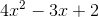Explanation: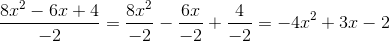### Example Question #6 : How To Divide Variables

Simplify: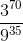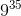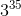Explanation:

To simplify, we want to get the same base in both the numerator and the denominator. This will allow us to easily manipulate the exponents.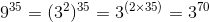Replace the denominator with this value and simplify.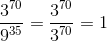### Example Question #1 : How To Divide Variables

Simplify: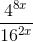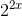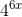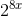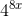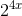Explanation:

To simplify, we want to get the same base in both the numerator and the denominator. This will allow us to easily manipulate the exponents.We can write: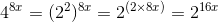and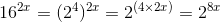Replace the fraction with these values and simplify.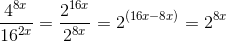### Example Question #2 : How To Divide Variables

Which of the following numbers is divisible by 7?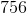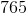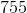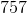Explanation:

The number 756 can be segmented into 700 and 56. Given that 700 is divisble by 7 (700 divided by 7 is 100) and that 56 is divisible by 7 (56 divided by 7 is 8), 756, as the aggregate of 700 and 56, is also divisble by 7.

### Example Question #9 : How To Divide Variables

What is the equivalent of this expression?

The square root of 64 divided by the square of 2.Explanation:

The mathematical translation of the square root of 64 divided by the square of 2 is: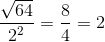### Example Question #10 : How To Divide Variables

Which of the following is divisible by 3?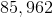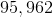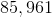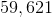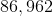Explanation:

A quick trick to remember is that a number is divisible by 3 when the digits of that number add up to a sum that is also divisible by 3.

For example, we know that the number 85,962 must be divisible by 3 because: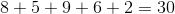30 is divisible by 3; therefore, 85,962 must also be divisible by 3.

In contrast, adding the digits of the other answer choices will give numbers that are not divisible by 3, indicating that the other answer choices are also not divisible by 3.

← Previous 1 3